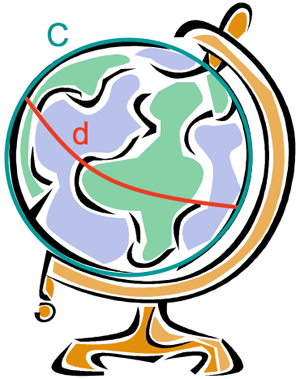## Tutorial 3: Circles in Curved Space

If you've worked through Tutorial 2: Triangles in Curved Space, then you know that the normal rules of Euclidean geometry no longer apply in curved space, so it shouldn't be too much of a surprise that the ratio between a circle's circumference and its diameter is no longer necessarily pi.Circle on a Globe
Again, go back to the example of a globe. The equator is an example of a circle on the surface of the globe; we know that a circle is defined as the set of points that are equidistant from a specified center point, and the equator represents all of the points that are the same distance from the North Pole. (Note that the equator also represents all of the points that are equidistant from the South Pole. Are all circles in this type of curved space dually defined like this?) Now think of the diameter of the equator; it would be along one of the longitude lines across the North Pole, and back down to the equator. The length of this line would be half the length of the equator, so the ratio of circumference to diameter in this case would be 2. The diagram shows another example of a circle on the surface of the globe; the circle is along the 90 degree east and west longitude lines, and the diameter is along the equator.

Verify this using the applet. To do this, first you must draw a great circle, or a circle that encompasses the entire sphere at its widest point. (Longitude lines are great circles on a globe, but latitude lines are generally not. Do you understand why?) Drawing a great circle in the applet is simple, you just move forward using the navigation buttons until you reach the point where you started. You probably want to turn the trail on first so you know where you've been. If you make sure to reset the odometer on the right side of the applet before you begin your journey, you'll have a method of measuring the length of your great circle.

Once you've walked out the great circle, and recorded the distance traveled, reset the odometer again, rotate 90 degrees in either direction, and walk across the great circle until you get to the other side. Record the distance walked across the circle, and find the ratio between the circumference and the diameter. Does this value agree with what we predicted using the spherical embedding model of the globe?

You can approximate other circles by moving forward a small distance and rotating through a small angle until you get back to where you started. For example, you can move forward 10 units, and rotate 10 degrees, and then repeat this process 36 times. Can you come up with other ways to approximate circles? Try making your own circle approximations and finding the ratio between their circumferences and diameters. Is there a limit on the value of this ratio in this type of curved space?

Copyright (C) 2010 Stephanie Erickson, Gary Felder
Terms and Conditions of Use